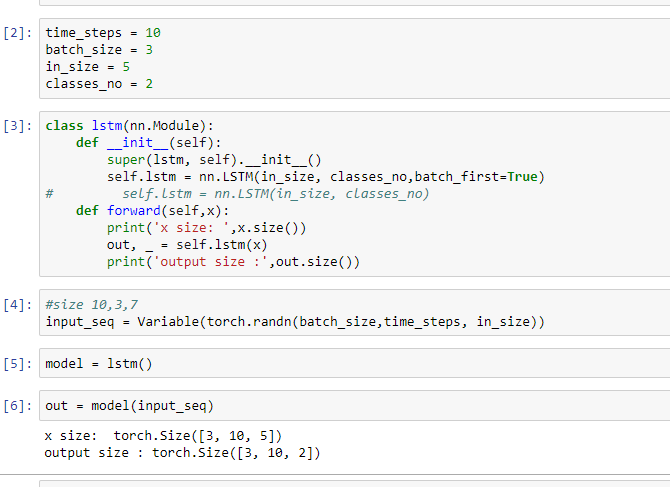# Could someone explain batch_first=True in LSTM

I can’t figure out how it works. I try to change bs,time step, input size with batch_first = True or no batch_first. It return the dimension that I feed to model. so I have to change it manually ??

2 Likes

If your input data is of shape `(seq_len, batch_size, features)` then you don’t need `batch_first=True` and your LSTM will give output of shape `(seq_len, batch_size, hidden_size)`.

If your input data is of shape `(batch_size, seq_len, features)` then you need `batch_first=True` and your LSTM will give output of shape `(batch_size, seq_len, hidden_size)`.

Is that not what you expect?

9 Likes

but when my input’s shape is `(batch_size, seq_len, features)` without `batch_first=True` the output still as same as the input that why i had a little doubt

``````no batch_first=True
``````

https://i.imgur.com/51SRQni.png

``````had batch_first=True
``````https://i.imgur.com/PI0Excd.png

If you feed it input of shape `(10, 20, in_features)` then the output will always be of shape `(10, 20, hidden_size)`

Without `batch_first=True` it will use the first dimension as the sequence dimension.
With `batch_first=True` it will use the second dimension as the sequence dimension.

It does not work the result should become 3x2

I really appreciate that your reply to all my topic thanks alot

When you do

``````print(out[-1])
``````

you are taking the last element of the batch dimension.

You probably wanted to do

``print(out[:, -1])``
3 Likes

Note that both version are only meaningful when `bidirectional=False`.